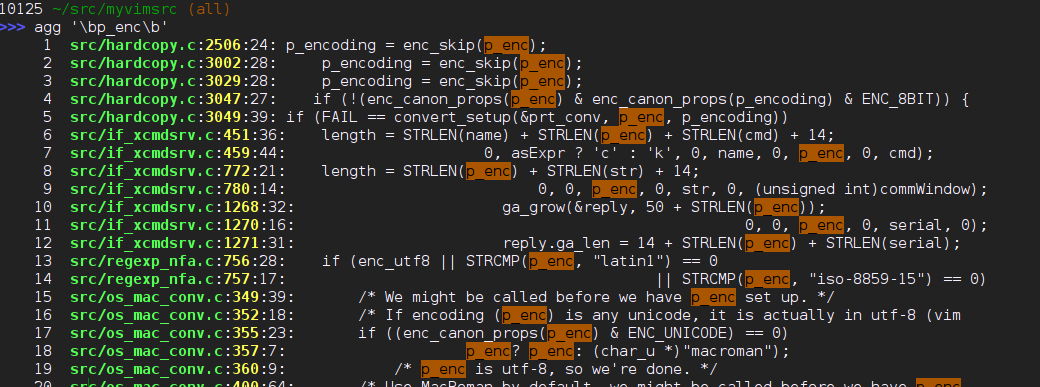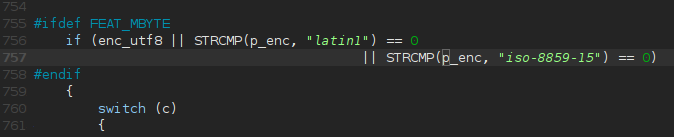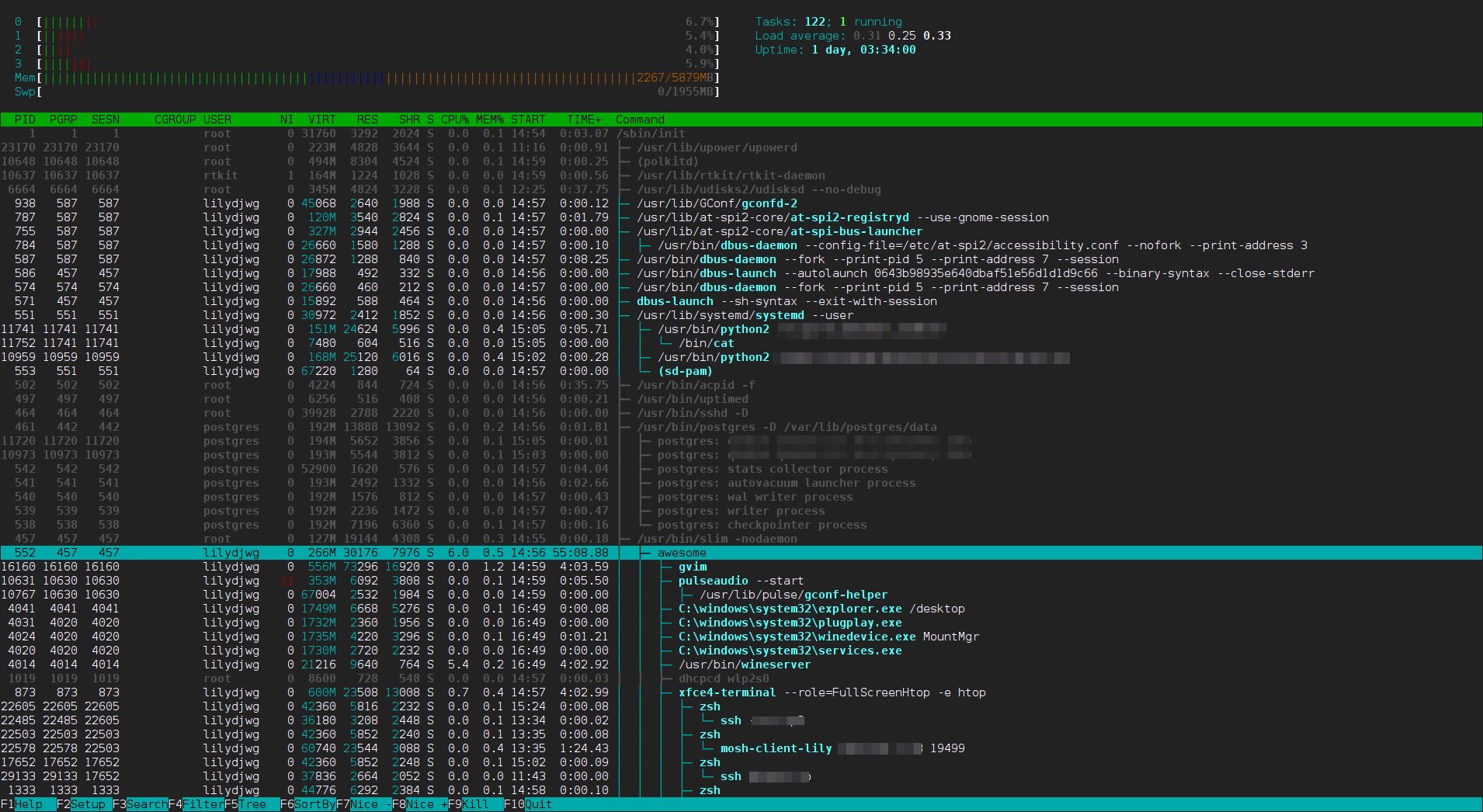8
5
2014

### 3. QR Code

```[abcdefghijklmnopqrstuvwxyz] <=> [pvwdgazxubqfsnrhocitlkeymj]
```

```>>> T = str.maketrans('pvwdgazxubqfsnrhocitlkeymj', 'abcdefghijklmnopqrstuvwxyz')
>>> s = 'Wxgcg txgcg ui p ixgff, txgcg ui p epm. I gyhgwt mrl lig txg ixgff wrsspnd tr irfkg txui hcrvfgs, nre, hfgpig tcm liunz txg crt13 ra "ixgff" t
r gntgc ngyt fgkgf.'
>>> s.translate(T)
'Where there is a shell, there is a way. I expect you use the shell command to solve this problem, now, please try using the rot13 of "shell" to enter next level.'
```

```rot13 <<< shell
```

Arch 上，rot13 命令位于 bsd-games 包。或者在 Vim 里把光标移动到「shell」单词上按g?aw也能得到结果。

### 4. cat

```%!grep -oP '(.)(.)(.)\2\1'
```

```v/\v^([A-Z][0-9]|[0-9][A-Z])\l/d
```

### 5. variables

```\$ n=1024
\$ while true; do n=\$(curl -sS http://fun.coolshell.cn/n/\$n); echo \$n; done
```

### 7. N Queens

```main = mapM_ print \$ queens 9
```

```\$ ./queens | tr -d ',[]' | while read code; do [[ \$(sha1sum <<<zWp8LGn01wxJ7\$code | awk '{print \$1}') == e48d316ed573d3273931e19f9ac9f9e6039a4242 ]] && echo \$code; done
```

### 8. Excel Column

26 进制转十进制：

```>>> def debase26(x):
...   return sum(26 ** i * (ord(d) - ord('A') + 1) for i, d in enumerate(x[::-1]))
...
>>> debase26('COOLSHELL') // base26('SHELL')
85165
```

```>>> def base64(x):
...   L = []
...   while True:
...     x, d = divmod(x, 26)
...     if d == 0: break
...     L.append(d)
...   return ''.join(chr(x + ord('A') - 1) for x in L[::-1])
...
>>> base64(85165)
'DUYO'
```

### 9. Fraternal Organisation

PS: 最近博客访问和评论速度都挺慢的，请见谅。

Category: Linux | Tags: linux python shell fun
7
18
2014

## 缘起

ag 的优势：

• 命令短
• 和 ack 以及 git grep 一样，默认会忽略掉你通常不想看的文本（二进制文件、被版本控制系统忽略掉的文件）
• C 编写的，比 ack 更快！

## 搜索agg 脚本更改了 ag 的显示样式，每一项开头都有一个序号，就和 cg 命令一样。同样地，agg 也把这个结果保存在主目录下的一个文件，以供 agv 命令使用。

## 使用编辑器打开

agv 命令不给定参数，会显示上一次搜索的结果。如果给出一个编号，就会将结果在`AGV_EDITOR`环境变量指定的编辑器里打开，并跳转到对应的地方。和 vg 不同的是，agg/agv 为每一个终端维护了一个结果列表，这样就可以在不同的终端里搜索不同的内容而不会相互干扰了。vv 需要 Python easygui 库，以及 gVim（或者 Vim）的 +clientserver 支持。vv 不仅支持上述格式的参数，作为一名 Pythonista 所编写的工具，它也支持从 Python 报错时打印的 Traceback 的行中提取文件名和行号。比如：

```vv 'File "/usr/lib/python3.4/sre_parse.py", line 358, in _parse_sub'
```

```alias -g XS='"\$(xsel)"'
```

```vv XS
```

## ag.vim

ag.vim 是一个 ack.vim 的修改版，用于在 Vim 中调用 ag，就像 ack.vim 在 Vim 中调用 ack、grep.vim 在 Vim 中调用 grep 一样。

```command Agg exe 'Ag -Q ' . expand('<cword>')
```

## 仓库地址

Category: Linux | Tags: python grep AG
6
17
2014

### Google IP 可用性检测脚本

```#!/usr/bin/env python3

import sys
from ipaddress import IPv4Network
import http.client as client
from concurrent.futures import ThreadPoolExecutor
import argparse
import ssl
import socket

# 先按自己的情况修改以下几行
APP_ID = 'your_id_here'
APP_PATH = '/fetch.py'

context = ssl.SSLContext(ssl.PROTOCOL_TLSv1)
context.verify_mode = ssl.CERT_REQUIRED

class HTTPSConnection(client.HTTPSConnection):
def __init__(self, *args, hostname=None, **kwargs):
self._hostname = hostname
super().__init__(*args, **kwargs)

def connect(self):
super(client.HTTPSConnection, self).connect()

if self._tunnel_host:
server_hostname = self._tunnel_host
else:
server_hostname = self._hostname or self.host
sni_hostname = server_hostname if ssl.HAS_SNI else None

self.sock = self._context.wrap_socket(self.sock,
server_hostname=sni_hostname)
if not self._context.check_hostname and self._check_hostname:
try:
ssl.match_hostname(self.sock.getpeercert(), server_hostname)
except Exception:
self.sock.shutdown(socket.SHUT_RDWR)
self.sock.close()
raise

def check_ip_p(ip, func):
if func(ip):
print(ip, flush=True)

def check_for_gae(ip):
return _check(APP_ID + '.appspot.com', APP_PATH, ip)

def check_for_search(ip):
return _check('www.google.com', '/', ip)

def _check(host, path, ip):
for chance in range(1,-1,-1):
try:
conn = HTTPSConnection(
ip, timeout = 5,
context = context,
hostname = host,
)
conn.request('GET', path, headers = {
'Host': host,
})
response = conn.getresponse()
if response.status < 400:
print('GOOD:', ip, file=sys.stderr)
else:
raise Exception('HTTP Error %s %s' % (
response.status, response.reason))
return True
except KeyboardInterrupt:
raise
except Exception as e:
if isinstance(e, ssl.CertificateError):
print('WARN: %s is not Google\'s!' % ip, file=sys.stderr)
chance = 0
if chance == 0:
print('BAD :', ip, e, file=sys.stderr)
return False
else:
print('RE  :', ip, e, file=sys.stderr)

def main():
parser = argparse.ArgumentParser(description='Check Google IPs')
help='service to check')
args = parser.parse_args()
func = globals()['check_for_' + args.service]

count = 0
with ThreadPoolExecutor(max_workers=50) as executor:
for l in sys.stdin:
l = l.strip()
if '/' in l:
for ip in IPv4Network(l).hosts():
executor.submit(check_ip_p, str(ip), func)
count += 1
else:
executor.submit(check_ip_p, l, func)
count += 1
print('%d IP checked.' % count)

if __name__ == '__main__':
main()
```

2014年9月3日重要更新：由于失误，之前的脚本没有检查 SSL/TLS 证书，所以将错误的 IP 认为是可用的。现已更新。

Category: python | Tags: python google 网络 中国特色
6
1
2014

### 给 Python 加上分数和十进制数字面量表示

```irb(main):001:0> 1/3r
=> (1/3)
irb(main):002:0> 1/3r+1/2r
=> (5/6)
```

```>>> from fractions import Fraction as R
>>> R(1, 3)
Fraction(1, 3)
>>> R(1, 3) + R(1, 2)
Fraction(5, 6)
```

```diff -r bf65e7db066d Parser/tokenizer.c
--- a/Parser/tokenizer.c    Mon Apr 14 22:27:27 2014 -0400
+++ b/Parser/tokenizer.c    Fri May 30 20:12:07 2014 +0800
@@ -1528,6 +1528,10 @@
goto fraction;
if (c == 'j' || c == 'J')
goto imaginary;
+            if (c == 'd' || c == 'D')
+                goto decimal;
+            if (c == 'r' || c == 'R')
+                goto rational;
if (c == 'x' || c == 'X') {

/* Hex */
@@ -1621,6 +1625,12 @@
/* Imaginary part */
imaginary:
c = tok_nextc(tok);
+                else if (c == 'd' || c == 'D')
+        decimal:
+                    c = tok_nextc(tok);
+                else if (c == 'r' || c == 'R')
+        rational:
+                    c = tok_nextc(tok);
}
}
tok_backup(tok, c);
```

`d` 后缀是我给十进制数——就是会计里会用到的精确的十进制小数——准备的。

```./configure --enable-shared --with-threads --with-computed-gotos --enable-ipv6 --with-valgrind --with-system-expat --with-dbmliborder=gdbm:ndbm --with-system-ffi --with-system-libmpdec --without-ensurepip
make
```

```LD_LIBRARY_PATH=. ./python
```

```>>> 4d
ValueError: could not convert string to float: 4d
```

```diff -r bf65e7db066d Python/ast.c
--- a/Python/ast.c  Mon Apr 14 22:27:27 2014 -0400
+++ b/Python/ast.c  Fri May 30 20:12:07 2014 +0800
@@ -3650,12 +3650,29 @@
long x;
double dx;
Py_complex compl;
-    int imflag;
+    char typeflag;
+    PyObject *mod, *type, *ret;

assert(s != NULL);
errno = 0;
end = s + strlen(s) - 1;
-    imflag = *end == 'j' || *end == 'J';
+    switch(*end){
+        case 'j':
+        case 'J':
+            typeflag = 'j';
+            break;
+        case 'd':
+        case 'D':
+            typeflag = 'd';
+            break;
+        case 'r':
+        case 'R':
+            typeflag = 'r';
+            break;
+        default:
+            typeflag = 'i';
+    }
+
if (s == '0') {
x = (long) PyOS_strtoul(s, (char **)&end, 0);
if (x < 0 && errno == 0) {
@@ -3670,13 +3687,43 @@
return PyLong_FromLong(x);
}
/* XXX Huge floats may silently fail */
-    if (imflag) {
+    if (typeflag == 'j') {
compl.real = 0.;
compl.imag = PyOS_string_to_double(s, (char **)&end, NULL);
if (compl.imag == -1.0 && PyErr_Occurred())
return NULL;
return PyComplex_FromCComplex(compl);
}
+    else if (typeflag == 'd') {
+      mod = PyImport_ImportModule("decimal");
+      if (mod == NULL)
+          return NULL;
+
+      type = PyObject_GetAttrString(mod, "Decimal");
+      if (type == NULL) {
+          Py_DECREF(mod);
+          return NULL;
+      }
+      ret = PyObject_CallFunction(type, "s#", s, strlen(s)-1);
+      Py_DECREF(type);
+      Py_DECREF(mod);
+      return ret;
+    }
+    else if (typeflag == 'r') {
+      mod = PyImport_ImportModule("fractions");
+      if (mod == NULL)
+          return NULL;
+
+      type = PyObject_GetAttrString(mod, "Fraction");
+      if (type == NULL) {
+          Py_DECREF(mod);
+          return NULL;
+      }
+      ret = PyObject_CallFunction(type, "s#", s, strlen(s)-1);
+      Py_DECREF(type);
+      Py_DECREF(mod);
+      return ret;
+    }
else
{
dx = PyOS_string_to_double(s, NULL, NULL);
```

```diff -r bf65e7db066d Lib/decimal.py
--- a/Lib/decimal.py    Mon Apr 14 22:27:27 2014 -0400
+++ b/Lib/decimal.py    Fri May 30 20:12:07 2014 +0800
@@ -1015,7 +1015,7 @@
def __repr__(self):
"""Represents the number as an instance of Decimal."""
# Invariant:  eval(repr(d)) == d
-        return "Decimal('%s')" % str(self)
+        return str(self) + 'd'

def __str__(self, eng=False, context=None):
"""Return string representation of the number in scientific notation.
diff -r bf65e7db066d Lib/fractions.py
--- a/Lib/fractions.py  Mon Apr 14 22:27:27 2014 -0400
+++ b/Lib/fractions.py  Fri May 30 20:12:07 2014 +0800
@@ -280,7 +280,7 @@

def __repr__(self):
"""repr(self)"""
-        return ('Fraction(%s, %s)' % (self._numerator, self._denominator))
+        return str(self) + 'r'

def __str__(self):
"""str(self)"""
diff -r bf65e7db066d Modules/_decimal/_decimal.c
--- a/Modules/_decimal/_decimal.c   Mon Apr 14 22:27:27 2014 -0400
+++ b/Modules/_decimal/_decimal.c   Fri May 30 20:12:07 2014 +0800
@@ -3092,18 +3092,10 @@
static PyObject *
dec_repr(PyObject *dec)
{
-    PyObject *res, *context;
-    char *cp;
-
-    CURRENT_CONTEXT(context);
-    cp = mpd_to_sci(MPD(dec), CtxCaps(context));
-    if (cp == NULL) {
-        PyErr_NoMemory();
-        return NULL;
-    }
-
-    res = PyUnicode_FromFormat("Decimal('%s')", cp);
-    mpd_free(cp);
+    PyObject *res, *str;
+    str = dec_str(dec);
+    res = PyUnicode_FromFormat("%Ud", str);
+    Py_DECREF(str);
return res;
}

```

```>>> 0.1 + 0.2 == 0.3
False
>>> 0.1d + 0.2d == 0.3d
True
>>> 1/3r + 1/2r
5/6r
>>> 0.4/1.2r
0.33333333333333337
>>> 0.4r/1.2r
1/3r
```

Category: python | Tags: Python C代码
5
27
2014

### 纯真 IP 数据库 QQWry 解析库 Python 3 版

2014年8月2日更新：增加了在线更新的功能，从此不需要 Wine 就能更新数据库啦 :-) 更新方法来自微菜。更新命令如下：

`python3 -m QQWry update`
Category: python | Tags: python IP地址
3
13
2014

### Python 3 的 super() 和 __class__

```class A:
def m(self):
print('A')

class B(A):
def m(self):
print('B')
super().m()

B().m()
```

```class B(A):
def m(self):
print('B')
super(B, self).m()
```

super() 很好地解决了访问父类中的方法的问题。那么，如果要访问父类的父类（准确地说，是方法解析顺序（MRO）中位于第三的类）的属性呢？

```class C(B):
def m(self):
print('C')
# see the difference!
print(__class__.__mro__)
print(self.__class__.__mro__)
__class__.__mro__.m(self)

class D(C):
def m(self):
print('D')
super().m()

o = D()
o.m()
```

```D
C
(<class 't.C'>, <class 't.B'>, <class 't.A'>, <class 'object'>)
(<class 't.D'>, <class 't.C'>, <class 't.B'>, <class 't.A'>, <class 'object'>)
A
```

Category: python | Tags: Python
3
6
2014

### 使用 PyQt 转换网页到 PDF

```#!/usr/bin/env python3

import sys

try:
from PyQt4 import QtWebKit
from PyQt4.QtCore import QUrl
from PyQt4.QtGui import QApplication, QPrinter
except ImportError:
from PySide import QtWebKit
from PySide.QtCore import QUrl
from PySide.QtGui import QApplication, QPrinter

app = QApplication(sys.argv)

def done(status):
p = QPrinter()
p.setOutputFormat(QPrinter.PdfFormat)
p.setOutputFileName('a.pdf')
view.print(p)
app.exit()

view = QtWebKit.QWebView()
# PySide does not have QApplication.exec
app.exec_()
```

Category: python | Tags: Python PyQt Qt
3
3
2014

### 《冰雪奇缘》之缘——A站弹幕 JSON 转 crt

you-get 竟助纣为虐，把歌词给下回来了！只叹 soimort 没有好人做到底，留一个语义不详的 JSON 让我情何以堪。

```#!/usr/bin/env python3

import json
import sys

for o in data:
c = o['c'].split(',')
m = o['m']
# see
# https://github.com/muzuiget/niconvert/blob/master/niconvert/libsite/acfun.py
start = float(c)
yield start, m

def format_time(t):
s, ms = divmod(t, 1)
s = int(s)
ms = int(ms * 1000)
m, s = divmod(s, 60)
h, m = divmod(m, 60)
return '%d:%02d:%02d,%03d' % (h, m, s, ms)

def crt_writer(f):
i = 0

fmt = '%d\n%s --> %s\n%s\n\n'
try:
while True:
t, m = yield
ts = format_time(t)
if i != 0:
f.write(fmt % (i, format_time(old_t), ts, old_m))
i += 1
old_t = t
old_m = m
except GeneratorExit:
f.write(fmt % (
i+1, format_time(t), format_time(t+2), m))

def main():
w = crt_writer(sys.stdout)
w.send(None)
old_d0 = 0
for d in cmtjson_reader(sys.stdin):
if d < old_d0:
break
w.send(d)
old_d0 = d

if __name__ == '__main__':
main()
```

mplayer 君虽识得 UTF-8 之码，却不通 fontconfig 之术，部分文字沦为了下划线。又及。

Category: python | Tags: python
2
23
2014

### 让我们收养孤儿进程吧

```        if (arg_running_as == SYSTEMD_USER) {
/* Become reaper of our children */
if (prctl(PR_SET_CHILD_SUBREAPER, 1) < 0) {
log_warning("Failed to make us a subreaper: %m");
if (errno == EINVAL)
log_info("Perhaps the kernel version is too old (< 3.4?)");
}
}
```

```#include<sys/prctl.h>
#include<Python.h>

static PyObject* subreap(PyObject *self, PyObject *args){
PyObject* pyreaping;
int reaping;
int result;

if (!PyArg_ParseTuple(args, "O!", &PyBool_Type, &pyreaping))
return NULL;
reaping = pyreaping == Py_True;

result = prctl(PR_SET_CHILD_SUBREAPER, reaping);

if(result != 0){
return PyErr_SetFromErrno(PyExc_OSError);
}else{
Py_RETURN_NONE;
}
}

static PyMethodDef mysysutil_methods[] = {
{"subreap", subreap, METH_VARARGS},
{NULL, NULL}    /* Sentinel */
};

static PyModuleDef mysysutil = {
"mysysutil",
"My system utils",
-1,
mysysutil_methods,
NULL, NULL, NULL, NULL
};

PyMODINIT_FUNC PyInit_mysysutil(void){
PyObject* m;

m = PyModule_Create(&mysysutil);
if(m == NULL)
return NULL;
return m;
}
```

```>>> import mysysutil
>>> mysysutil.subreap(True)
```

```#include<lua.h>
#include<lualib.h>
#include<lauxlib.h>

#include<sys/prctl.h>
#include<sys/wait.h>
#include<errno.h>
#include<string.h>
#include<signal.h>

static int l_setsubreap(lua_State * L){
int reap;
if(lua_isboolean(L, 1)){
reap = lua_toboolean(L, 1);
}else{
return luaL_argerror(L, 1, "not a boolean");
}
if(prctl(PR_SET_CHILD_SUBREAPER, reap) != 0){
return luaL_error(L, "prctl failed: %s", strerror(errno));
}
return 0;
}

static int l_ignore_SIGCHLD(lua_State * L){
signal(SIGCHLD, SIG_IGN);
return 0;
}

static int l_reap(lua_State * L){
int pid, st;
pid = waitpid(-1, &st, WNOHANG);
lua_pushinteger(L, st);
lua_pushinteger(L, pid);
return 2;
}

static const struct luaL_Reg l_lib[] = {
{"setsubreap", l_setsubreap},
{"reap", l_reap},
{"ignore_SIGCHLD", l_ignore_SIGCHLD},
{NULL, NULL}
};

int luaopen_clua(lua_State * L){
lua_newtable(L);
luaL_setfuncs(L, l_lib, 0);
return 1;
}
```

```package.cpath = package.cpath .. ';/home/lilydjwg/scripts/lua/cmod/?.so'
clua = require('clua')
clua.setsubreap(true)
clua.ignore_SIGCHLD()
```1
15
2014

### 被 Tornado coroutine 对异常的异常支持坑了

```>>> python -m this | grep -A1 -F Errors
Errors should never pass silently.
Unless explicitly silenced.
```

```from tornado.gen import coroutine, Task
from tornado.process import Subprocess

@coroutine
def run_cmd(cmd):
p = Subprocess(
cmd,
stdout = Subprocess.STREAM,
stderr = Subprocess.STREAM,
)
return out, err, code
# For Python below 3.3, use
# raise Return((out, err, code))
```

yield 一个 Task（或者 Future）的列表的话，它们会并发执行，全部执行完毕之后才会返回到这个 yield 位置继续执行。简洁干净。（不过我要吐槽一下为什么必须传列表，传元组就不对……）

```#!/usr/bin/env python3

from tornado.gen import coroutine
from tornado.ioloop import IOLoop

@coroutine
def two():
print('two entered')
1 / 0
print('two leaving')

@coroutine
def one():
print('one entered')
yield two()
print('one leaving')

if __name__ == '__main__':
one()
IOLoop.current().start()
```

```one entered
two entered
```

```#!/usr/bin/env python3

from tornado.gen import coroutine
from tornado.ioloop import IOLoop

@coroutine
def two():
print('two entered')
1 / 0
print('two leaving')

@coroutine
def one():
print('one entered')
yield two()
print('one leaving')

def _future_done(fu):
fu.result()

if __name__ == '__main__':
fu = one()
IOLoop.current().start()
```

```one entered
two entered
ERROR:concurrent.futures:exception calling callback for <Future at 0x7f286c0bcf90 state=finished raised ZeroDivisionError>
...
File "t.py", line 9, in two
1 / 0
ZeroDivisionError: division by zero
```

2014年8月2日更新：asyncio 在遇到这种情况时会打印错误日志，参见文档

Category: python | Tags: python tornado coroutine

| Theme: Aeros 2.0 by TheBuckmaker.com